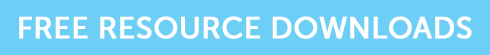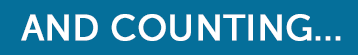Correlation is key to diversification, but what does it mean?

# Correlation is key to diversification, but what does it mean?

We are often told that correlation is a key aspect of diversification, but what does it mean? This article explains what correlation is and provides an example of a table that could appear on the CII R02 exam – also useful for CII J10, AF4, R06 and AF5 revision.

### What is correlation?

Correlation is a measure of dependency between asset classes or investments.  Correlation varies between plus 1 and minus 1.  If assets are positively correlated, then their returns move in the same direction (either up or down).  When assets have negative correlation, their returns move in opposite directions.  No correlation means there is no dependency, so each investment very much does its own thing.

### Effective Diversification

The most effective diversification comes from investments that are negatively correlated.  Perfectly negatively correlated assets move in the opposite direction from each other by the same amount.  These would provide 100% diversification, as there would be no variance and therefore, no risk.  However, it would be very difficult to find investments that work like this.  You’ll know that correlation between asset classes rose during the financial crisis, but historically, property and cash, you could say, are negatively correlated asset classes and would provide good diversification in a portfolio. With no correlation, the movement of two investments’ prices are totally unrelated, i.e., they are independent of each other so having a few investments with near on zero correlation would also provide good diversification.

### A Typical CII R02 Exam Question

Here is a typical question from the R02 exam.  You could get a table very much like this one and then need to analyse the information in order to answer the question.The manual doesn’t really adequately explain how this table works, so basically it is saying that asset X and Y have relatively high correlation (0.8); asset X and Z have negative correlation (-0.2) and Y and Z have low correlation (0.3).  For example, if Asset X were to rise by say 8%, then asset Y would rise by 80% of that figure, ie 6.4%.

### Grab the resources you need!

If you’re studying for your CII R02 exam, and you’re seeking some extra practice, grab our free taster to try out one of Brand Financial Training’s resources for yourself.  Click the link to download the R02 calculation workbook taster now!Alternatively, you can download taster resources for AF4AF5, J10 or R06 if you’re revising for one of those exams.

### Over to You…

Can you think of an example where assets are positively correlated?